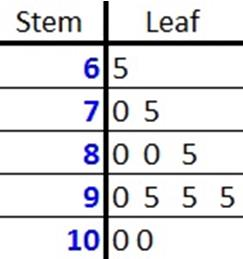© Ms. Garcia Math 7th The stem-and- leaf plot below shows test scores in Math. GR36 answersUse the stem and leaf plot to answer these questions. 1) What is the best test score? 2) What is the lowest score? 100 65 Answer Answer 3) What is the difference between the high and low scores? 4) How many students scored 70? 35 1 Answer Answer Name: ID: